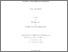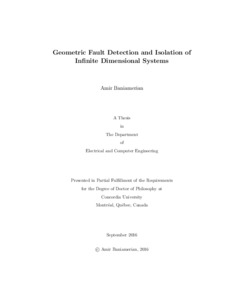Title:

# Geometric Fault Detection and Isolation of Infinite Dimensional Systems

Baniamerian, Amir (2016) Geometric Fault Detection and Isolation of Infinite Dimensional Systems. PhD thesis, Concordia University.Preview
Text (application/pdf)
Baniamerian_PhD_F2016.pdf - Accepted Version
1MB

## Abstract

A broad class of dynamical systems from chemical processes to flexible mechanical structures, heat transfer and compression processes in gas turbine engines are represented by a set of partial differential equations (PDE). These systems are known as infinite dimensional (Inf-D) systems. Most of Inf-D systems, including PDEs and time-delayed systems can be represented by a differential equation in an appropriate Hilbert space. These Hilbert spaces are essentially Inf-D vector spaces, and therefore, they are utilized to represent Inf-D dynamical systems. Inf-D systems have been investigated by invoking two schemes, namely approximate and exact methods. Both approaches extend the control theory of ordinary differential equation (ODE) systems to Inf-D systems, however by utilizing two different methodologies. In the former approach, one needs to first approximate the original Inf-D system by an ODE system (e.g. by using finite element or finite difference methods) and then apply the established control theory of ODEs to the approximated model. On the other hand, in the exact approach, one investigates the Inf-D system without using any approximation. In other words, one first represents the system as an Inf-D system and then investigates it in the corresponding Inf-D Hilbert space by extending and generalizing the available results of finite-dimensional (Fin-D) control theory.

It is well-known that one of the challenging issues in control theory is development of algorithms such that the controlled system can maintain the required performance even in presence of faults. In the literature, this property is known as fault tolerant control. The fault detection and isolation (FDI) analysis is the first step in order to achieve this goal. For Inf-D systems, the currently available results on the FDI problem are quite limited and restricted. This thesis is mainly concerned with the FDI problem of the linear Inf-D systems by using both approximate and exact approaches based on the geometric control theory of Fin-D and Inf-D systems. This thesis addresses this problem by developing a geometric FDI framework for Inf-D systems. Moreover, we implement and demonstrate a methodology for applying our results to mathematical models of a heat transfer and a two-component reaction-diffusion processes.

In this thesis, we first investigate the development of an FDI scheme for discrete-time multi-dimensional (nD) systems that represent approximate models for Inf-D systems. The basic invariant subspaces including unobservable and unobservability subspaces of one-dimensional (1D) systems are extended to nD models. Sufficient conditions for solvability of the FDI problem are provided, where an LMI-based approach is also derived for the observer design. The capability of our proposed FDI methodology is demonstrated through numerical simulation results to an approximation of a hyperbolic partial differential equation system of a heat exchanger that is represented as a two-dimensional (2D) system.

In the second part, an FDI methodology for the Riesz spectral (RS) system is investigated. RS systems represent a large class of parabolic and hyperbolic PDE in Inf-D systems framework. This part is mainly concerned with the equivalence of different types of invariant subspaces as defined for RS systems. Necessary and sufficient conditions for solvability of the FDI problem are developed. Moreover, for a subclass of RS systems, we first provide algorithms (for computing the invariant subspaces) that converge in a finite and known number of steps and then derive the necessary and sufficient conditions for solvability of the FDI problem.

Finally, by generalizing the results that are developed for RS systems necessary and sufficient conditions for solvability of the FDI problem in a general Inf-D system are derived. Particularly, we first address invariant subspaces of Fin-D systems from a new point of view by invoking resolvent operators. This approach enables one to extend the previous Fin-D results to Inf-D systems. Particularly, necessary and sufficient conditions for equivalence of various types of conditioned and controlled invariant subspaces of Inf-D systems are obtained. Duality properties of Inf-D systems are then investigated. By introducing unobservability subspaces for Inf-D systems the FDI problem is formally formulated, and necessary and sufficient conditions for solvability of the FDI problem are provided.

Divisions: Concordia University > Gina Cody School of Engineering and Computer Science > Electrical and Computer Engineering Thesis (PhD) Baniamerian, Amir Concordia University Ph. D. Electrical and Computer Engineering 12 September 2016 Khorasani, Khashayar and Meskin, Nader Geometric Fault Detection and Isolation, Multidimensional Systems, Infinite Dimensional systems, Invariant Subspaces, 981764 AMIR BANIAMERIAN 09 Nov 2016 15:19 18 Jan 2018 17:53
All items in Spectrum are protected by copyright, with all rights reserved. The use of items is governed by Spectrum's terms of access.

Repository Staff Only: item control pageResearch related to the current document (at the CORE website)
Back to top